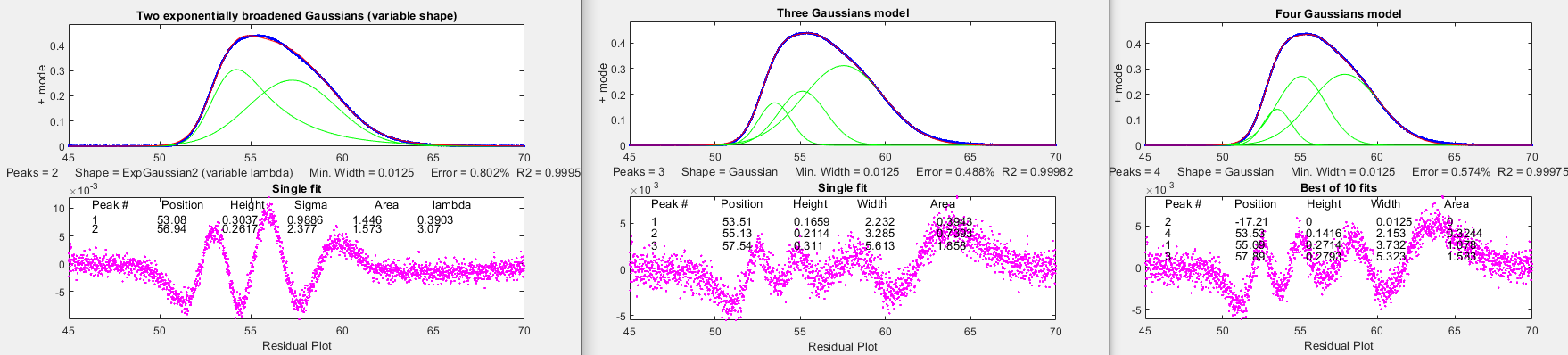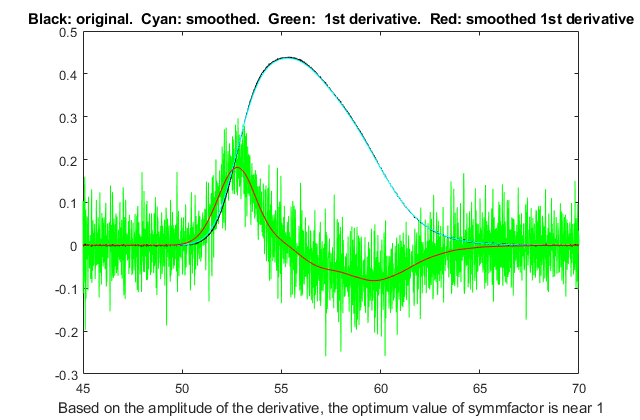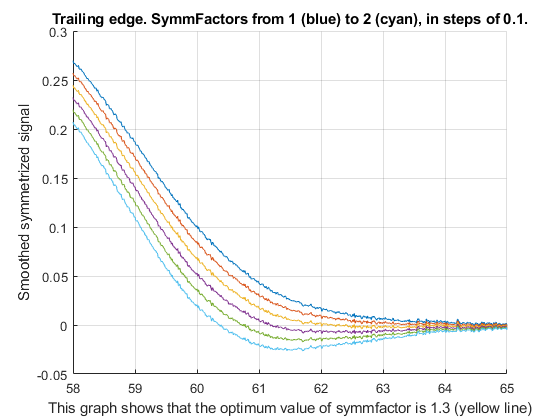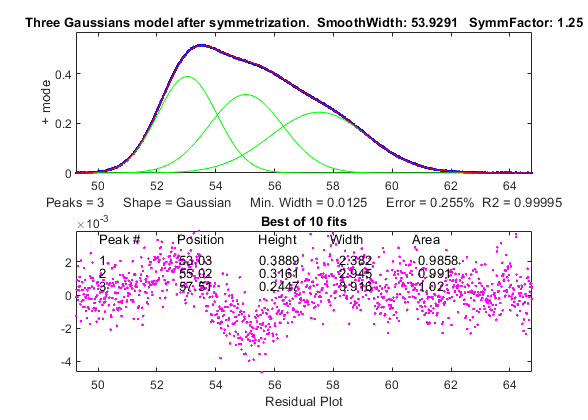[Introduction]  [Signal arithmetic]  [Signals and noise]   [Smoothing]   [Differentiation]  [Peak Sharpening]  [Harmonic analysis]   [Fourier convolution]  [Fourier deconvolution]  [Fourier filter]  [Wavelets]   [Peak area measurement]  [Linear Least Squares]  [Multicomponent Spectroscopy]  [Iterative Curve Fitting]  [Hyperlinear quantitative absorption spectrophotometry] [Appendix and Case Studies]  [Peak Finding and Measurement]  [iPeak]   [iSignal]  [Peak Fitters]   [iFilter]  [iPower]  [List of downloadable software]  [Interactive tools]

## Appendix AE. The mystery peak challenge

The objective of this exercise is to learn as much as we can about the underlying properties of a digitized signal using the signal processing tools in this book and, if possible, to obtain a mathematical description of the signal (script). At first glance, the signal (MysteryPeak.mat) appears to be a single, asymmetrical peak with a maximum at x=55.5. The signal-to-noise ratio seems to be very good - there is little visible noise unless you look very closely - and the signal begins and ends near zero, so baseline correction is likely not an issue. The bad news is that we do not know anything else. The asymmetry might be due to some asymmetrical process applied to an originally symmetrical peak shape, but it could be a group of closely spaced overlapping peaks, which is suggested by the faint bumps in the shape. Some quick preliminary curve fitting can be done using command line peakfit.m:

for a two-Gaussian model (shape 1)
[FitResults,GOF]=peakfit([x y],0,0,2,1)

for a single Logistic model (shape 3)

[FitResults,GOF]=peakfit([x y],0,0,1,3)

for a 4 exponentially broadened Gaussian model (shape 39)
[FitResults,GOF]=peakfit([x y],0,0,4,39,1)Alternatively, it is even easier to use the interactive peak fitter
ipf.m for this purpose; you can quickly change the model shape, number of peaks, starting guesses, data region to be fitted, etc., with single keystrokes and mouse clicks. Either way, the three initial fits in the figures show that the signal contains a small amount of random noise, which appears to be white (so the signal has probably not been smoothed, which is fortunate) and which has a relative standard deviation of about 0.2%, based on 1/5th of the visual peak-to-peak value in the residual plot. But unfortunately these fits are not successful because their fitting errors (0.5 to 0.8%) are all significantly larger than the 0.2% random noise. Trying different shapes and greater numbers of peaks does not help either, resulting in either higher fitting errors, unstable fits, or zero peak heights for additional peaks (as in the four-Gaussian fit).Another approach to the problem of asymmetrical peaks is to use the technique of first-derivative symmetrization described previously. This applies specifically to exponential broadening, a commonly- encountered peak broadening mechanism. The idea is that if you compute the first derivative of an exponentially broadened peak, multiply it by a weighting factor equal to the time constant of the exponential, and add it to the original broadened signal, the result will be the original peak before broadening, which makes the peak overlap less severe. This works for any original peak shape. Even if you do not know the time constant beforehand, you can try different values until the baseline after the peak is as low as possible but not negative, as shown in this GIF animation. This is easily done interactively in iSignal, version 7, which has smoothing, derivatives, symmetrization, and curve fitting, or by using the symmetrize.m function. The derivative of y with respect to x, by the "derivxy.m" function, shown by the green line in the figure above, is quite noisy. As usual, we must smooth the derivatives of noisy signals to make them useful, but we must not over-smooth and distort the signals. As a rule of thumb, a smooth width equal to 1/10th of the number of data points in the halfwidth does not distort the signal visibly.Our peak has about 530 points (
=halfwidth(1:length(y),y)), so a smooth width near 53 will not distort the signal peak, but it does eliminate most of the noise from the derivative (red line, above right). Also, we can see that the derivative, in y/x units, is comparable in numerical magnitude to the original signal, so the weighting factor (in x units) is probably somewhere near 1.0. Next, we add the first derivative times that factor to the original signal, looking at the trailing edge as we try six different factor values near 1.0. The graph on the left shows that the optimum value is about 1.25.

When this symmetrization is applied to the entire signal, the result, shown belowon the right, has more distinct bumps. When that modified signal is used for curve fitting, it is found that a 3-Gaussian model works quite well, with a fitting error of only 0.25%. This is evidence that the signal actually consists of three exponentially broadened Gaussians. Ordinarily there is no independent way to check the accuracy of the peak parameters so measured, but - full disclosure - the signal in the case was not actually unknown but rather was generated by the file MysteryPeaks.m. The true time constant is 1.3, the peak positions are 53, 55, and 57.5 and the peak areas are each equal to 1.0. The curve-fit results after symmetrization (shown  on the right) are within 0.1% for the peak positions and within 2% of the areas. In contrast, direct fitting of three equal-alpha exponential Gaussians to the original data, which is now possible because we have determined a value for the time constant
(expressed as the number of data points: symmfactor./(x(2)-x(1)), gives less accurate results in this case (graphic), probably because the overlap of the asymmetrical peak is greater. Moreover, it takes three times longer to compute.

This page is part of "A Pragmatic Introduction to Signal Processing", created and maintained by Tom O'Haver, Professor Emeritus, Department of Chemistry and Biochemistry, The University of Maryland at College Park. Comments, suggestions and questions should be directed to Prof. O'Haver at toh@umd.edu. Updated July, 2022.